# Pendulum Project

Inverted pendulum is mainly used as benchmark for testing new control algorithms. In the scope of Bavarian Graduate School of Computational Engineering Honors Project (BGCE) we developed classical controller using mathematical description of the inverted pendulum system and a fuzzy logic based controller. We have successfully developed a simulation model and implemented the above mentioned controllers on both the simulation and real system. We have also developed easy-to-use and informative graphical user interface (GUI) with visualization of the simulated system, state variable response diagram, and fuzzy rule control. All the controller functionalities for both the simulation and real system have been integrated into the GUI. Also, we have been able to implement Backpropagation based learning algorithm for the adaptive neural controller and obtain satisfactory preliminary results.

## Introduction

An inverted pendulum is a pendulum which is attached to a cart. (Fig. 1.1)

Even though a regular inverted pendulum has a point mass attached to its rod, our hardware consists of a cart which is free to move horizontally and a rod attached to it. An inverted pendulum is inherently unstable, and must be actively balanced in order to remain upright, either by applying a torque at the pivot point or by moving the pivot point horizontally as part of a feedback system. 

In control theory and classical dynamics the inverted pendulum is considered to be a classic problem which is used as benchmark for testing of the control algorithms. As some examples of the control algorithms we used the inverted pendulum system to experiment with PID controller, fuzzy controller and lastly neural networks.

Besides its theoretical importance the inverted pendulum system has also seen action in real life. An inverted pendulum-like system is used during the take-offs of the rocket to keep the rocket in balance. Moreover, the shipping cranes in the seaports use a similar idea. Last but not the least, a self-balancing transportation device called Segway uses same working principle as it is in an inverted pendulum.

## BGCE Project Scope

The classical approach of control theory is to develop a mathematical model for the system and then to develop a corresponding controller so that the closed loop is stable (PID- controller). A second approach is the use of a so-called fuzzy-controller, which doesn’t need an explicit model of the system. Another interesting possibility is to use self-learning controllers that learn automatically how to control the system. Techniques such as neural networks can be used for this task. In the scope of this project, these different approaches will be studied. The focus is not only on the controllers themselves, but also on their visualization and demonstration via an interactive graphical user interface.

## Classical Controller

Classical Controller tries to solve the physical equations governing the system in order to find the trajectory of the rod from the stable equilibrium position at rest to the unstable equilibrium position.

To achieve this goal we first have to find the mathematical representation of the system, meaning , we will model the system at first and then we try to find the relation between the output of the system which is the current state as feed back and the next input which hopefully helps us get closer to the unstable equilibrium position.

### Classical Model

The purpose of system modeling is to provide an accurate mathematical description of the system. Once this description is obtained, the numerical constants of the system will be substituted into the equations to provide a working representation of the system. From there this model can be simulated and manipulated to obtain gain values on which the state feedback control is based.

This section will derive two sets of mathematical models of the system. The first model is a set of nonlinear differential equations. The second model is a set of linearized differential state equations.

Due to the difficulty level of nonlinear control and the scope of this project, the nonlinear equations will not be used for control calculations. However, the linearized equations provide a good estimate of the system under conditions mentioned in this chapter, and will be used for all control calculations.

Since the inverted pendulum is a complex system, the more accurate one wants to be the more complex the model gets and the slower the controller.

So having this fact on mind , we tried to use the most important terms and leave away the parts where we thought wouldn't be much of importance but of course doing this we moved away from a complex model to an easier to solve one in cost of accuracy.

The physical model of the inverted pendulum on a moving cart is seen in figure 1. The inverted pendulum system is free to move only in the X-Y plane. The symbolic descriptions are shown in table 3.1.

Using the physical model of the inverted pendulum from Fig. 1, we can now derive the differential equations that describe the system as seen in the following section.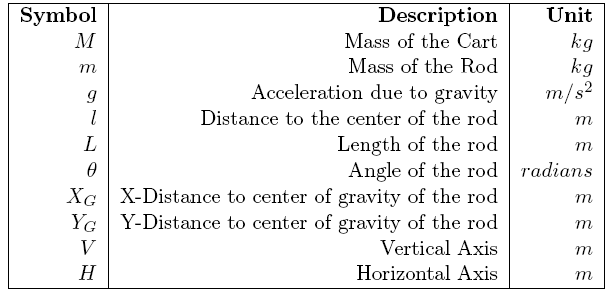Table 3.1.1 Parameters of the Model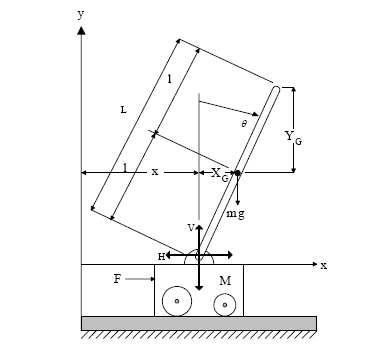Fig. 3.1.1 Inverted Pendulum Model

in progress...

### Controller Design

The self-erecting inverted pendulum has a control design for the swing up, and a separate control design for the stabilization. The open loop swing up controller brings the pendulum upright close to the unstable point of equilibrium. Once the angular position has reached a software adjustable capture range, the closed loop stabilization controller takes over.

#### Closed Loop Stabilization Control Design

The stabilization control design is based on linear quadratic regulator (LQR) design with a tracking controller. The objective of this controller is to stabilize the pendulum rod in the upright unstable point of equilibrium while maintaining a software adjustable linear set point position. The LQR design will effectively return the state feedback gains needed to ensure stability of the system. However, to bring the steady state error of the linear position to zero, a tracking controller is added by integrating the error of the cart position, relative to the linear set point, over time. The gain adjustment of the integration result allows control over the zero steady state error convergence time.

Fig. 3.5.2.1 Simulink Control Design Block Diagram

## Fuzzy Controller

### Introduction

Instead of the mathematical modeling and writing differential equations describing the characteristics of the inverted pendulum system another controller technique named "Fuzzy Controller" is introduced initially to mimic human behavior in robotics. Since the human body is perfect in keeping its balance, the scientists came up with the inverted pendulum system to represent the human-like balancing as closest as it could be. To actualize this idea the fuzzy logic and fuzzy controllers are used to stabilize the inverted pendulum with its rod kept upright.

### Fuzzy Logic

Fuzzy logic (FL) is a form of multi-valued logic derived from fuzzy set theory to deal with reasoning that is approximate rather than precise. Just as in fuzzy set theory the set membership values can range (inclusively) between 0 and 1, in fuzzy logic the degree of truth of a statement can range between 0 and 1 and is not constrained to the two truth values {true (1), false (0)} as in classic predicate logic.

FL provides a simple way to arrive at a definite conclusion based upon vague, ambiguous, imprecise, noisy, or missing input information. FL's approach to control problems mimics how a person would make decisions, only much faster.

### Distinctive Properties of Fuzzy Logic

FL incorporates a simple, rule-based IF X AND Y THEN Z approach in order to a solve control problem rather than attempting to model a system mathematically. The FL model is empirically-based, relying on an operator's experience rather than their technical understanding of the system. For example, rather than dealing with terms such as exact values (x=100 or 50<x<100), terms like "IF (x is too big) AND (x is getting smaller) THEN (push the cart)" etc. These terms are imprecise and yet very descriptive of what must actually happen. Similar style is used while describing the inverted pendulum system.

### Fuzzy Logic in Inverted Pendulum

In the inverted pendulum system we defined four state variables: position of the cart (s), velocity of the cart (sp), angular velocity of the rod(phip) and angle of the rod(phi_oben). Those state variables play a big role when the subcontroller "stabilizer" kicks in to keep the pendulum stable in the neighborhood of the 0 degree. Each state variable is divided into several membership functions shown as in the Fig. 4.4.1.

$\textstyle phip$ and $\textstyle phi\_oben$ are divided into 7 states categorized as: $\textstyle negative\_big$, $\textstyle negative$, $\textstyle negative\_small$,$\textstyle zero$, $\textstyle positive_small$, $\textstyle positive$, $\textstyle positive\_big$. Each category is associated with a range of values. The ranges were:

Angle: [-0.3, 0.3]

Angular Velocity: [-4, 4]

Position: [-0.4,0.4]

Velocity: [-0.5, 0.5]

At each time step those four state variables are computed and a fuzzy rule corresponding to that specific state of the system will become active. Thus, it makes the cart move in one direction as it is specified in that fuzzy rule.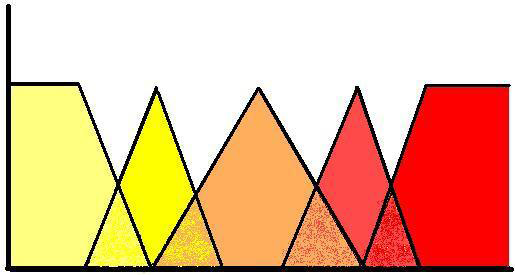Fig. 4.4.1 Membership Function Example

In Fig. 4.4.1 above, we can see five membership functions made up by triangles (except the left-and rightmost areas).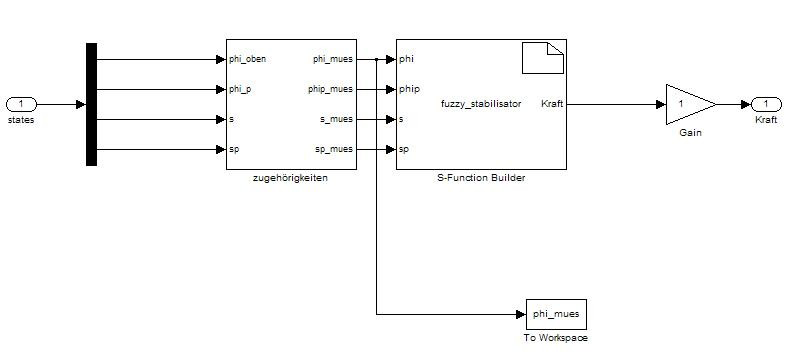Fig. 4.4.2 Simulink Model Diagram of the Stabilizer

The erector swings the rod like a sine-wave and aims to bring the rod close to the region ($\textstyle +/- 0.3^\circ$) so that the stabilizer can kick in. Fig. 4.5.4 above depicts the inside of the stabilizer. The membership functions are defined in "zugehörigkeiten" block whereas the fuzzy rules are defined inside the S-Function builder called "fuzzy_stabilisator".

### Results

The fuzzy controller (FC) has produced during our implementation better results than the initial implementation. When the project started, we had a version of a fuzzy controller. The main problem with that FC was that the swinger part was slow and it took too much time for till the stabilizer kicked in. Our main focus was to implement a better swing-up controller and to improve the current set of fuzzy rules. However, our experiments even with new fuzzy rules did not improve the stabilizer. Hence, we kept the old fuzzy rules defined in the beginning of the project.

In our trials, we implemented Gaussian membership functions to get a smoother fuzzy controller acting on the inverted pendulum. Yet, we did not see any significant gain in terms of speedup and robustness of the pendulum system. That is why we decide rolled back those membership functions to their initial states where they are represented as triangles.

## Neural Networks

The characteristics of the inverted pendulum make identification and control more challenging, as we have seen from the above sections. This can be circumvented by using a neural network based identification-control approach ,,. This would involve first, developing an accurate model of the inverted pendulum system using neural networks – System Identification. Then, develop a neural network controller which determines the correct control action to stabilize the system.

### Artificial Neural Networks

The science of artificial neural networks is based on the neuron. In order to understand the structure of artificial networks, the basic elements of the neuron should be understood. Neurons are the fundamental elements in the central nervous system. Fig. 5.1 below depicts the components of a neuron .Fig. 5.1 Components of a Neuron

A neuron is made up of 3 main parts -dendrites, cell body and axon. The dendrites receive signals coming from the neighbouring neurons. The dendrites send their signals to the body of the cell. The cell body contains the nucleus of the neuron. If the sum of the received signals is greater than a threshold value, the neuron fires by sending an electrical pulse along the axon to the next neuron.

The following model is based on the components of the biological neuron. The inputs X0-X3 represent the dendrites. Each input is multiplied by weights W0, W3. The output of the neuron model, Y is a function, F of the summation of the input signals. (Fig. 5.2)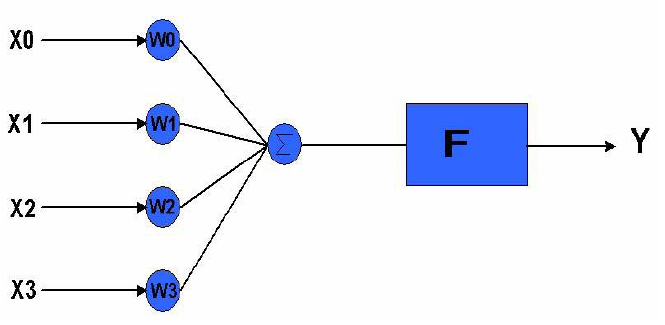Fig. 5.2 Dentrides

The main advantage of ANN is they operate as black boxes. This avoids most of the complex modeling activities, and reduces the model to just a set of weights. But, this also would be a disadvantage since the rules of operation in neural networks are completely unknown. But the disadvantage is the amount of time taken to train networks. It can take considerable time to train an ANN for certain functions.

### Neural Network Structures

There are 3 main types of ANN structures -single layer feed-forward network, multi-layer feed-forward network and recurrent networks . In feed-forward networks the direction of signals is from input to output, there is no feedback in the layers. Other types of single layer networks are based on the perceptron model. The details of the perceptron are shown in Fig. 5.2.1.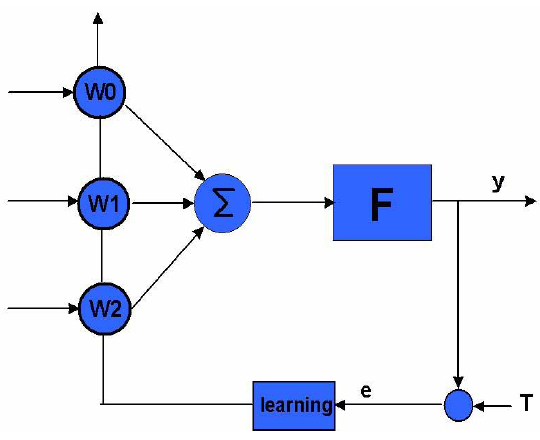Fig. 5.2.1 Perceptron

In the current work, we employ a multi-layer perceptron model with a single hidden layer.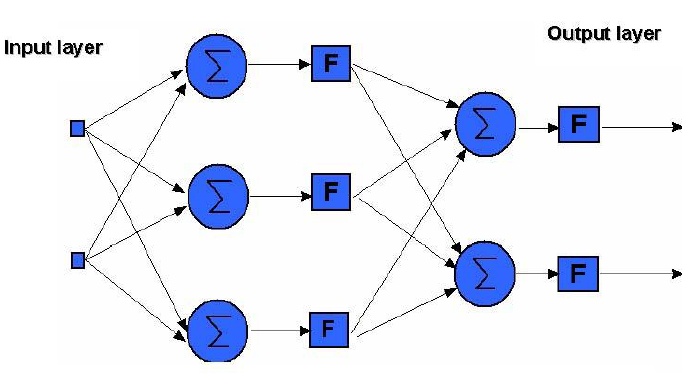Fig. 5.2.2 Three Layer Neural Network Structure

Increasing the number of neurons in the hidden layer or adding more hidden layers to the network allows the network to deal with more complex functions. Cybenko's theorem states that, "A feed-forward neural network with a sufficiently large number of hidden neurons with continuous and differentiable transfer functions can approximate any continuous function over a closed interval.".  The weights in MLP's are updated using the back-propagation learning algorithm , which is discussed in detail in the coming sections.

Inputs to the perceptron are individually weighted and then summed. The perceptron computes the output as a function $\textstyle F$ of the sum. The activation function, $\textstyle F$ is needed to introduce nonlinearities into the network. This makes multi-layer networks powerful in representing nonlinear functions. There are 3 main types of activation function -tan-sigmoid, log-sigmoid and linear . Different activation functions affect the performance of an ANN.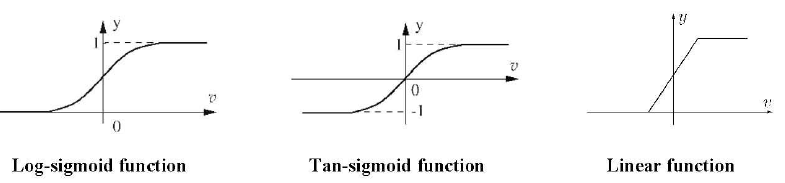Fig. 5.2.3 Activation Functions

The output from the perceptron is

$\textstyle y[k] = f (w [k].x[k])$.

### ANN Learning

Neural networks have 3 main modes of operation – supervised, reinforced and unsupervised learning . In supervised learning the output from the neural network is compared with a set of targets, the error signal is used to update the weights in the neural network. Reinforced learning is similar to supervised learning however there are no targets given, the algorithm is given a grade of the ANN performance. Unsupervised learning updates the weights based on the input data only. The ANN learns to cluster different input patterns into different classes.

### 5.4 Back-propagation Learning Algorithm

Back-propagation Learning Algorithm is a supervised learning method, and is an implementation of the Delta rule . As the name itself suggests, the errors are propagate backwards from the output nodes to the inner nodes and therefore the learning. So back-propagation is used to calculate the gradient of the error of the network with respect to the network's modifiable weights. This gradient is almost always then used in a simple stochastic gradient descent algorithm to find weights that minimize the error. But, the term "back-propagation" is used in a more general sense, and refers to the entire procedure encompassing both the calculation of the gradient and its use in stochastic gradient descent. Back-propagation usually allows quick convergence on satisfactory local minima for error in the kind of networks to which it is suited. But, this also brings out its inherent drawback since it usually converges to the local minima, instead of the global minima.

There are two passes before the weights are updated. In the first pass (forward pass) the outputs of all neurons are calculated by multiplying the input vector by the weights. The error is calculated for each of the output layer neurons. In the backward pass, the error is passed back through the network layer by layer. The weights are adjusted according to the gradient decent rule, so that the actual output of the MLP moves closer to the desired output. A momentum term could be added which increases the learning rate with stability.

During the (second) backward pass, the difference between the target output and the actual output (error) is calculated

$\textstyle e[k]=T[k] - y[k]$.

The errors are back propagated through the layers and the weight changes are made. The formula for adjusting the weights is

$\textstyle w[k+1] = w[k]+\mu e[k]x[k].$

Once the weights are adjusted, the feed-forward process is repeated. The weights are adapted until the error between the target and actual output is low. The approximation of the function improves as the error decreases.

The Algorithm can be summarized as below:

1. Present a training sample to the neural network.
2. Compare the network's output to the desired output from that sample. Calculate the error in each output neuron.
3. For each neuron, calculate what the output should have been, and a scaling factor, how much lower or higher the output must be adjusted to match the desired output. This is the local error.
4. Adjust the weights of each neuron to lower the local error.
5. Assign an inertial value for the local error to neurons at the previous level, giving greater responsibility to neurons connected by stronger weights.
6. Repeat from step 3 on the neurons at the previous level, using each one's inertial value as its error.

### System Identification

System identification is the process of developing a mathematical model of a dynamic system based on the input and output data from the actual process . This means it is possible to sample the input and output signals of a system and using this data generate a mathematical model. An important stage in control system design is the development of a mathematical model of the system to be controlled. In order to develop a controller, it must be possible to analyze the system to be controlled and this is done using a mathematical model. Another advantage of system identification is evident if the process is changed or modified. System identification allows the real system to be altered without having to calculate the dynamical equations and model the parameters again. This circumvents most of the mathematically rigorous modeling activities involved in case of complex real systems. The mathematical model in this case is the black box, it describes the relationship between the input and output signals. The inverted pendulum system is a non-linear process. To adequately model it,non-linear methods using neural networks must be used. It can be seen from the literature that neural networks have been successful used in modeling a plethora of nonlinear systems.

As universal approximators, neural networks have found widespread application in nonlinear dynamic system identification.. The most common method of neural network identification is called forward modeling. During training both the process and ANN receive the same input, the outputs from the ANN and process are compared, this error signal is used to update the weights in the ANN. This is an example of supervised learning-the teacher (pendulum system) provides target values for the learner (the neural network).

\includegraphics[width=0.5\textwidth]{supervised_learning.PNG} \textbf{Fig. 5.5.1} Supervised Learning

This training/identification can also be done off-line, having collected the input-output data from the inverted pendulum system. In the current work, for the input-output data for the identification of the model the non-linear pendulum model along with a classical feedback LQR controller(discussed in previous sections), is used.

### Specifics of the ANN Training

The quality of the neural model is tested by calculating the MSE (mean squared error). The MSE gives a good indication of the accuracy of the model. The MSE between the model and the process should be low. A model could have a low MSE but not predict any of the dynamics of the pendulum system. The output from the model and process is plotted to compare the dynamics. Basically, we want to see whether the model predicts the movement of the inverted pendulum. Increasing the number of hidden layer neurons allows for more complex functions to be modeled. During testing, neural networks with a range of hidden layer neurons were simulated. It was expected that as the number of hidden neurons increased the more accurate the model would become.

In progress..

## Graphical User Interface

This Pendulum GUI is a friendly graphical user interface for the BGCE Inverted Pendulum Project. You can easily switch into Classical controller, Fuzzy controller or Neural network by choosing the different Tab of this GUI, also can change between Simulation or Real-time. By default, the Classical controller mode. (Fig. 6.1)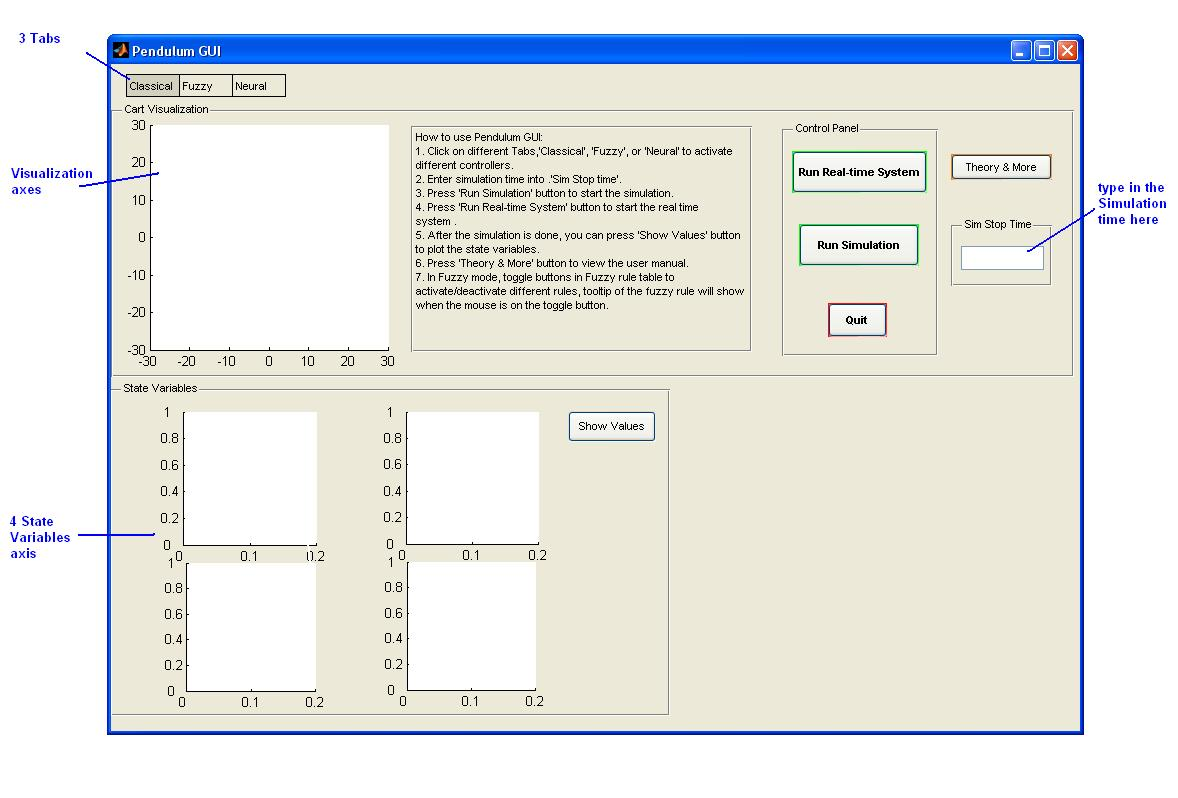Fig. 6.1 Default Pendulum GUI (Classical controller mode)

### Classical Controller Tab

The "Classical" tab consists of Cart Visualization panel, Control panel and State Variables panel.

-Simulation
First, type the required simulation time into Sim Stop Time text box, then click the Run Simulation button to start the simulation. The visualization of the pendulum will be showed in the Visualization axes. After the simulation is done, click the Show Values button in the State Variables panel, the plot of 4 state variables will be showed in the State Variables axis.

-Real-time System
Click the \textbf{Run Real-time System} button to start the real-time system by the pre- complied .wcp file.

### Fuzzy Controller Tab

The layout of Fuzzy Tab based on the classical tab but added a Fuzzy rule table panel. (Fig. 6.2.1)

-Simulation
Same as Classical Tab. Because of the features of fuzzy controller, one can also control the fuzzy rules by press/depress the toggle buttons of the Fuzzy rule table. There are tool tips for all the toggle buttons, implies the rule of each button. All the rules are activated by default, deactivate the rule by pressing the related button(turn red), activate by depressing(turn green). The membership function of the fuzzy rules will also be plotted in the sub-GUI during the simulation.

-Real-time System
Same as Classical Tab.Fig. 6.2.1 Fuzzy controller simulation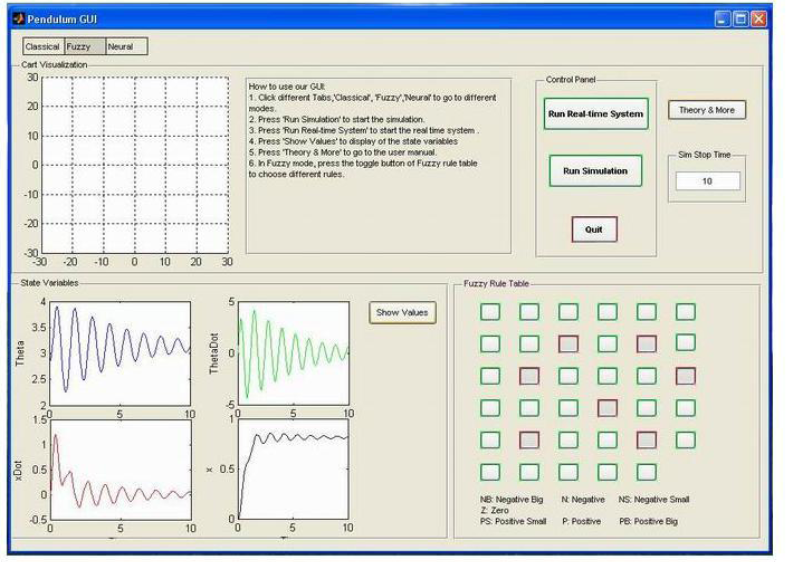Fig. 6.2.2 State Variables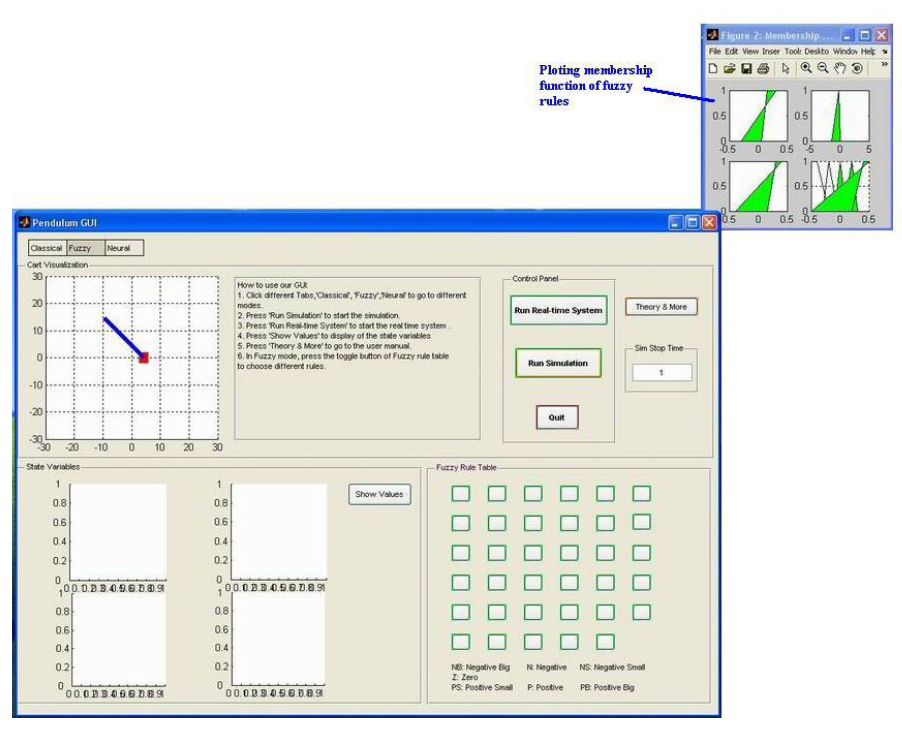Fig. 6.2.3 Membership Function of Fuzzy Rules

### Neural Networks Controller Tab

-Simulation
Same as Classical Tab.

-Real-time System
Same as Classical Tab.